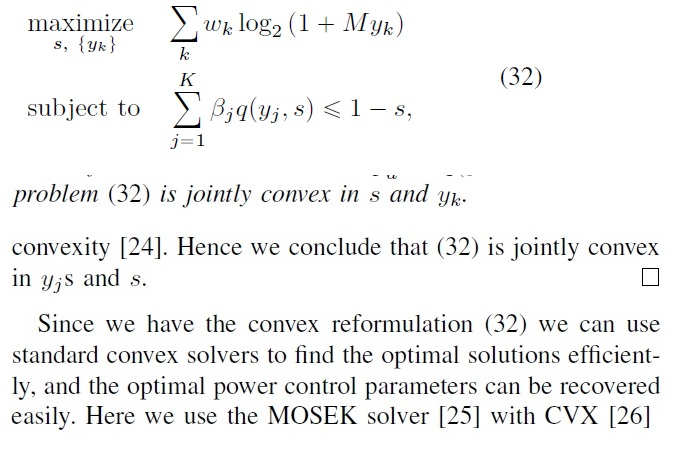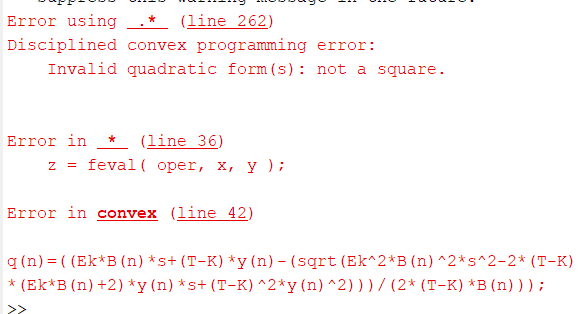# How can calculation this problem

How to write this Matlab code

my code:
cvx_begin
variable y(n) ;
variable s ;

for n=1:K
S=log(1+M*y(n));
SUM=SUM+S;
end

for n=1:K

``````q(n)=((Ek*B(n)*s+(T-K)*y(n)-(sqrt(Ek^2*B(n)^2*s^2-2*(T-K)*(Ek*B(n)+2)*y(n)*s+(T-K)^2*y(n)^2)))/(2*(T-K)*B(n)));
qq(n)=B(n)*q(n);
ST=ST+qq(n);
``````

end

maximize(SUM) ;
subject to
ST <= (1-s);
cvx_end

and error is :
Error using .* (line 262)
Disciplined convex programming error:
Invalid quadratic form(s): not a square.

Error in * (line 36)
z = feval( oper, x, y );

Error in convex (line 42)
q(n)=((EkB(n)s+(T-K)y(n)-(sqrt(Ek^2B(n)^2s^2-2(T-K)(EkB(n)+2)y(n)s+(T-K)^2y(n)^2)))/(2(T-K)*B(n)));

Do you have a proof that the constraint is convex (some kind of second order cone thing?)? I will presume it is non-convex unless you show otherwise, in which case the non-convex designation will be removed (edit: the non-convex designation has now been removed).

Per the CVX Users’ Guide and Why isn’t CVX accepting my model? READ THIS FIRST! , you are not allowed to enter the constraint as you have. And if it is somehow convex, reformulation will be required for entry into CVX.

is jointly convex and proof in the papera problem (32) is jointly convex y_j * s and s
a problem (32) is jointly convex y_j and s
and finally solved by MOSEK cvx

or

maximize(sum(log(1+M.*y)/log(2))) ;
subject to

sum(((Ek B(n). s+(T-K). y-(sqrt(Ek^2 B(n)^2. s^2-2 (T-K) (Ek B(n)+2).*y(n).*s+(T-K)^2. y(n)^2)))/(2 (T-K)))) <= (1-s);The paper appears to be https://arxiv.org/pdf/1612.02574.pdf , for which there was a later IEEE publication to which I don’t have access.

Even presuming the joint convexity proof (in the transformed variables) is correct, there is no concrete formulation provided in a form suitable for entry into CVX. I suggest you contact the authors and request them to provide you with their CVX code and allow you to post if here for the benefit of forum readers.